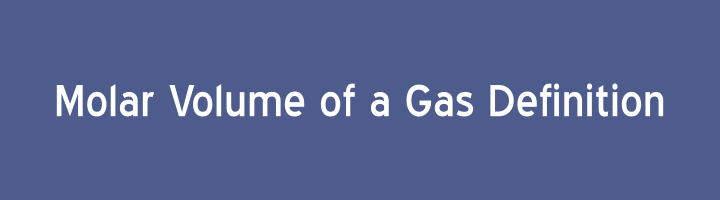# Molar Volume of a Gas DefinitionThe volume of one mole of an ideal gas at standard temperature and pressure is called molar volume of a gas and is equal to 22.414 dm3 at STP (Standard temperature & pressure) & 24 dm3 at RTP (Room temperature & pressure).

## Explanation:

At standard temperature and pressure:

2 g of hydrogen (H2) = 1 mole of hydrogen = 6.02 x 1023 molecules = 22.414 dm3

32 g of oxygen (O2) = 1 mole of oxygen = 6.02 x 1023 molecules = 22.414 dm3

16 g of methane (CH4) = 1 mole of methane = 6.02×1023 molecules = 22.414 dm3

Above data shows that volume of equal number of molecules of different gases at same temperature and pressure is equal. The mass and size of the molecule of the gas has no effect on the volume of the gas.